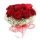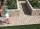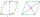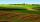# Flowerbed 2

Around the square flower bed in a park is sidewalk 2 m wide. The area of ​​this sidewalk is 243 m2. What is the area of the flowerbed?

S =  805.1 m2

### Step-by-step explanation:Did you find an error or inaccuracy? Feel free to write us. Thank you!#### You need to know the following knowledge to solve this word math problem:

We encourage you to watch this tutorial video on this math problem:

## Related math problems and questions:

• Flower bedThe park has a rectangular flower bed with dimensions of 3.2m and 1.5m. How many rose bushes will we plant in the flower bed if 20 dm2 are needed for one bush?
• SidewalkThe city park is a circular bed of flowers with a diameter of 8 meters. Around it, the whole length is 1-meter wide sidewalk. What is the sidewalk area?
• AroundAround the circular flowerbed with a radius of 2 m is a sidewalk 80 cm wide. How many square meters does the sidewalk have?
• The areaThe area of a rhombus is 143 m2. If the longer diagonal is 26 m, find the shorter diagonal in inches named d1.
• FlowerbedWe enlarge the circular flower bed, so its radius increased by 3 m. The substrate consumption per enlarged flower bed was (at the same layer height as before magnification) nine times greater than before. Determine the original flowerbed radius.
• The schoolyardThe schoolyard had the shape of a square with an 11m side. The yard has been enlarged by 75 m2 and has a square shape again. How many meters was each side of the yard enlarged?
• The sides 2The sides of a trapezoid are in the ratio 2:5:8:5. The trapezoid's area is 245. Find the height and the perimeter of the trapezoid.
• Trapezoid 15Area of trapezoid is 266. What value is x if bases b1 is 2x-3, b2 is 2x+1 and height h is x+4
• Land areasTwo land areas are 244 m2. The first parcel is 40 m2 less than twice the second one. What has the acreage of each parcel?
• Evaluate expressionEvaluate following expression: 5 to the power of 2 - (4x4) divided by 2 =
• Extending square gardenMrs. Petrová's garden had the shape of a square with a side length of 15 m. After its enlargement by 64 m2 (square), it had the shape of a square again. How many meters has the length of each side of the garden been extended?
• Six paintersSix painters paint an area of 120 m2 in 4 hours. How long does it take for 12 painters to paint 480 m2 with the same performance?
• The cubeThe cube has a surface area of 486 m ^ 2. Calculate its volume.
• Tripled squareIf you tripled the length of the sides of the square ABCD you increases its content by 200 cm2. How long is the side of the square ABCD?
• Sphere A2VThe surface of the sphere is 241 mm2. What is its volume?
• The cubeThe cube has a surface area of 216 dm2. Calculate: a) the content of one wall, b) edge length, c) cube volume.
• Circular lawnAround a circular lawn area is 2 m wide sidewalk. The outer edge of the sidewalk is curb whose width is 2 m. Curbstone and the inner side of the sidewalk together form a concentric circles. Calculate the area of the circular lawn and the result round to 1